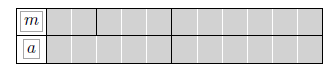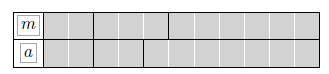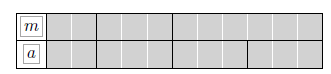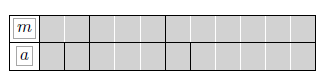# 任务定义

1. In Xanadu did Kubla Khan
2. A stately pleasure-dome decree:
3. Where Alph, the sacred river, ran
4. Through caverns measureless to man
5. Down to a sunless sea.
6. So twice five miles of fertile ground
7. With walls and towers were girdled round:
8. And here were gardens bright with sinuous rills,
9. Where blossomed many an incense-bearing tree;
10. And here were forests ancient as the hills,
11. Enfolding sunny spots of greenery.

1–2 Kubla Khan and his decree
3–5 Waterways
6–11 Fertile ground and greenery

# 评估指标

1. PRF值
这个评判标准在信息抽取（IR）中非常广泛的使用。在之前的文章中，我们就已经介绍过，这里不再赘述。

2. 基于分词的方法
基于这个方法有两种，一种是比较宽容的，以BMEO四个标签的正确与否来进行判别，另一类则是比较严格的，必须预测正确每一个span的首位置和尾位置才算正确，例如正确的span划分为(1,3),(4,4),(5,7)，那么你要是划分为(1,2),(3,4),(5,7)，则只算对1个。

3. $P_k$
$P_k$值最早在1997年TextTiling: Segmenting Text into Multi-paragraph Subtopic Passages 的论文中出现，后来在1999年Statistical Models for Text Segmentation中有了明确的定义，下面是它最原始的定义。
$P_μ(ref,hyp)=\sum_{1 \le i \le j \le n}D_μ(i,j)\delta_{ref}(i,j) XNOR \delta_{hpy}(i,j)$
我们不解释其公式的具体含义，我们解释一下它的目的，它就是使用一个窗口大小为K的滑动窗口（其中K如果不指定，K为标准分割的每个块的大小的平均值的一半），判断窗口的2个边缘的节点是否属于同一个主题，然后再看标准的判断和预测的判断是否一致即可，最后将一致的数量除以滑动次数就得出 $P_k$值。下面我们会有真实的例子进行讲解。

4. WindowDiff(WD)
从刚才的描述来看， $P_k$是一个相当麻烦的评估方法，而且并没有直接评估划分的是否正确，因此，到了2002年的论文（A Critique and Improvement of an Evaluation Metric for Text Segmentation），才有了Windowdiff(WD)。
WD指出Pk拥有5个问题：

1. False negatives penalized more than false positives（假负例比假正例更容易受到惩罚）
2. Number of boundaries ignored（忽略边界数量）
3. Sensitivity to variations in segment size (对文本块大小敏感)
4. Near-miss error penalized too much（对于小错误处罚太多）
5. What do the numbers mean? （指标意图不明显）

Windowdiff是替代Pk的评价指标，更加客观，其评估的公式为：
$WindowDiff (ref , hyp) = \frac{1}{N-k}\sum_{i=1}^{N-k}(|b(ref_i, ref_{i+k} ) − b(hyp_i, hyp_{i+k} )| > 0 )$
这个就比较直观，就是直接判别两个区域内的分割线数量的异同即可，其中K的定义与之前 $P_k$的一样。

5. Segmentation Similarity(S)
到了10年后，2012年Segmentation Similarity and Agreement使用编辑距离来更好的获取一些那种微小的错误（near misses）所带来的性能偏差。
$S(s_a,s_b,n_t)=1- \frac{|edits(s_a,s_b,n_t)|}{|pb(D)|}$
学过编辑距离的，就知道如何计算 $s_a$ $s_b$序列的编辑距离，pb(D)是潜在的边界数量，不知道的话，这里有传送门

6. Boundary Similarity (B)
然而就在1年以后，更先进的计算方法又出现了。在2013年的论文Evaluating Text Segmentation using Boundary Edit Distance中说，之前的3种方法都是具有偏见性的，并列举了4个例子（我们将会在下一部分见到）来说明新的评估方法的优势，下面是它的计算公式。
$B(s_1,s_2,n_t)=1-\frac{|A_e|+w_t\_span(T_e,n_t)+w_s\_ord(S_e,T_b)}{|A_e|+|T_e|+|S_e|+|B_m|}$
公式的具体细节可以看一下论文，论文里将的比较细，因为这不是重点，我们不再这重复讲解了。当然，我们也会在下面的示例中展示。从公式中，我们可以看到很多来源于S的想法，没错，其实这篇文章与2012的文章是同一个作者所写，他在之前的工作中进一步的进行了研究，并提出了一个更好的办法，但这并不能说明之前的工作没有意义。

# 实例说明# 代码实现

• mass
聚类的形式，一个列表包含每个部分的篇章单元的数目。
• postion
这就是一个连续的序列，并将每一个主题都进行了标号划分。
• boundary_string
这是一个连续篇章单元的二值化的结果，值得注意的是，这个结果虽然使用1表示该篇章单元为分割边界，但是并不在最后一个篇章单元上标志为1。
	# mass format [2, 3, 6]
masses = [2, 3, 6]
# position format (1, 1, 2, 2, 2, 3, 3, 3, 3, 3, 3)
positions = convert_masses_to_positions(masses)
# boundary_string ({0},{1},{0},{0},{1},{0},{0},{0},{0},{0})
boundary_string = boundary_string_from_masses(masses)


from segeval.window.pk import pk
from segeval.window.windowdiff import window_diff as WD
from segeval.similarity.boundary import boundary_similarity as B
from segeval.similarity.segmentation import segmentation_similarity as S

if __name__ == '__main__':
gold = [2, 3, 6]
h_list = [[5, 6], [2, 2, 7], [2, 3, 3, 3], [1, 1, 3, 1, 5]]
for n, h in enumerate(h_list):
print("第%d次实验" % int(n + 1))
print("Pk=%.3f, WD=%.3f, B=%.3f, S=%.3f" % (pk(h, gold, one_minus=True),
WD(h, gold, one_minus=True, window_size=2), B(h, gold), S(h, gold)))


第1次实验
Pk=0.778, WD=0.778, B=0.500, S=0.900

Pk=0.778, WD=0.778, B=0.750, S=0.950

Pk=0.778, WD=0.778, B=0.667, S=0.900

Pk=0.889, WD=0.667, B=0.500, S=0.800


PS: 对于完整的评估代码，我已经开源至Github中，代码链接